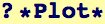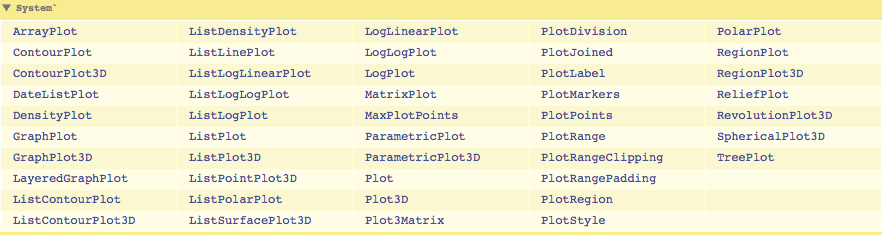Graphics and Plotting

Mathematica has a large number of built-in graphics programs for plotting and exploring mathematics graphically and for plotting and visualizing data.  Graphs and plots are effective ways of conveying complex information.  It is important to learn how to create and manipulate plots and graphics.  Mathematica is very rich in its graphical functions—we will explore just a small subset of all its capabilities.  We can get an idea of how many plotting routines are available by using a wildcard:While Mathematica has a large number of plotting routines, no one program does everything we need.  It can be useful to export numbers and graphics from Mathematica and operate on them individually or with other specialized programs.  The number of different creative graphical solutions available grows geometrically with the number of different graphical tools that are mastered.
Here, we will just produce a number of examples of plots and graphics...

 Created by Wolfram Mathematica 6.0  (28 August 2007)